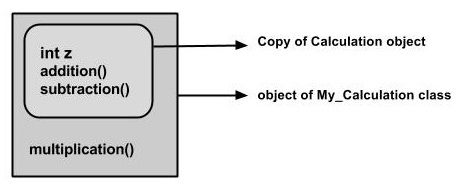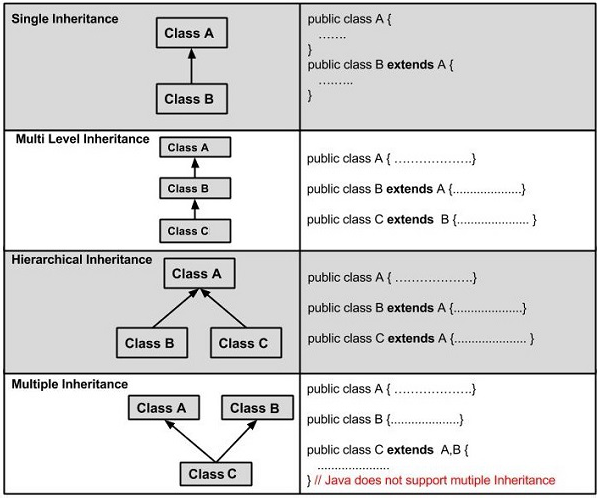# Java - Inheritance

Inheritance can be defined as the process where one class acquires the properties (methods and fields) of another. With the use of inheritance the information is made manageable in a hierarchical order.

The class which inherits the properties of other is known as subclass (derived class, child class) and the class whose properties are inherited is known as superclass (base class, parent class).

## extends Keyword

extends is the keyword used to inherit the properties of a class. Following is the syntax of extends keyword.

Syntax

```class Super {
.....
.....
}
class Sub extends Super {
.....
.....
}
```

## Sample Code

Following is an example demonstrating Java inheritance. In this example, you can observe two classes namely Calculation and My_Calculation.

Using extends keyword, the My_Calculation inherits the methods addition() and Subtraction() of Calculation class.

Copy and paste the following program in a file with name My_Calculation.java

Example

```class Calculation {
int z;

public void addition(int x, int y) {
z = x + y;
System.out.println("The sum of the given numbers:"+z);
}

public void Subtraction(int x, int y) {
z = x - y;
System.out.println("The difference between the given numbers:"+z);
}
}

public class My_Calculation extends Calculation {
public void multiplication(int x, int y) {
z = x * y;
System.out.println("The product of the given numbers:"+z);
}

public static void main(String args[]) {
int a = 20, b = 10;
My_Calculation demo = new My_Calculation();
demo.Subtraction(a, b);
demo.multiplication(a, b);
}
}
```

Compile and execute the above code as shown below.

```javac My_Calculation.java
java My_Calculation
```

After executing the program, it will produce the following result −

Output

```The sum of the given numbers:30
The difference between the given numbers:10
The product of the given numbers:200
```

In the given program, when an object to My_Calculation class is created, a copy of the contents of the superclass is made within it. That is why, using the object of the subclass you can access the members of a superclass.The Superclass reference variable can hold the subclass object, but using that variable you can access only the members of the superclass, so to access the members of both classes it is recommended to always create reference variable to the subclass.

If you consider the above program, you can instantiate the class as given below. But using the superclass reference variable ( cal in this case) you cannot call the method multiplication(), which belongs to the subclass My_Calculation.

```Calculation demo = new My_Calculation();
demo.Subtraction(a, b);
```

Note − A subclass inherits all the members (fields, methods, and nested classes) from its superclass. Constructors are not members, so they are not inherited by subclasses, but the constructor of the superclass can be invoked from the subclass.

## The super keyword

The super keyword is similar to this keyword. Following are the scenarios where the super keyword is used.

• It is used to differentiate the members of superclass from the members of subclass, if they have same names.

• It is used to invoke the superclass constructor from subclass.

### Differentiating the Members

If a class is inheriting the properties of another class. And if the members of the superclass have the names same as the sub class, to differentiate these variables we use super keyword as shown below.

```super.variable
super.method();
```

### Sample Code

This section provides you a program that demonstrates the usage of the super keyword.

In the given program, you have two classes namely Sub_class and Super_class, both have a method named display() with different implementations, and a variable named num with different values. We are invoking display() method of both classes and printing the value of the variable num of both classes. Here you can observe that we have used super keyword to differentiate the members of superclass from subclass.

Copy and paste the program in a file with name Sub_class.java.

Example

```class Super_class {
int num = 20;

// display method of superclass
public void display() {
System.out.println("This is the display method of superclass");
}
}

public class Sub_class extends Super_class {
int num = 10;

// display method of sub class
public void display() {
System.out.println("This is the display method of subclass");
}

public void my_method() {
// Instantiating subclass
Sub_class sub = new Sub_class();

// Invoking the display() method of sub class
sub.display();

// Invoking the display() method of superclass
super.display();

// printing the value of variable num of subclass
System.out.println("value of the variable named num in sub class:"+ sub.num);

// printing the value of variable num of superclass
System.out.println("value of the variable named num in super class:"+ super.num);
}

public static void main(String args[]) {
Sub_class obj = new Sub_class();
obj.my_method();
}
}
```

Compile and execute the above code using the following syntax.

```javac Super_Demo
java Super
```

On executing the program, you will get the following result −

Output

```This is the display method of subclass
This is the display method of superclass
value of the variable named num in sub class:10
value of the variable named num in super class:20
```

## Invoking Superclass Constructor

If a class is inheriting the properties of another class, the subclass automatically acquires the default constructor of the superclass. But if you want to call a parameterized constructor of the superclass, you need to use the super keyword as shown below.

```super(values);
```

### Sample Code

The program given in this section demonstrates how to use the super keyword to invoke the parametrized constructor of the superclass. This program contains a superclass and a subclass, where the superclass contains a parameterized constructor which accepts a integer value, and we used the super keyword to invoke the parameterized constructor of the superclass.

Copy and paste the following program in a file with the name Subclass.java

Example

```class Superclass {
int age;

Superclass(int age) {
this.age = age;
}

public void getAge() {
System.out.println("The value of the variable named age in super class is: " +age);
}
}

public class Subclass extends Superclass {
Subclass(int age) {
super(age);
}

public static void main(String args[]) {
Subclass s = new Subclass(24);
s.getAge();
}
}
```

Compile and execute the above code using the following syntax.

```javac Subclass
java Subclass
```

On executing the program, you will get the following result −

Output

```The value of the variable named age in super class is: 24
```

## IS-A Relationship

IS-A is a way of saying: This object is a type of that object. Let us see how the extends keyword is used to achieve inheritance.

```public class Animal {
}

public class Mammal extends Animal {
}

public class Reptile extends Animal {
}

public class Dog extends Mammal {
}
```

Now, based on the above example, in Object-Oriented terms, the following are true −

• Animal is the superclass of Mammal class.
• Animal is the superclass of Reptile class.
• Mammal and Reptile are subclasses of Animal class.
• Dog is the subclass of both Mammal and Animal classes.

Now, if we consider the IS-A relationship, we can say −

• Mammal IS-A Animal
• Reptile IS-A Animal
• Dog IS-A Mammal
• Hence: Dog IS-A Animal as well

With the use of the extends keyword, the subclasses will be able to inherit all the properties of the superclass except for the private properties of the superclass.

We can assure that Mammal is actually an Animal with the use of the instance operator.

Example

```class Animal {
}

class Mammal extends Animal {
}

class Reptile extends Animal {
}

public class Dog extends Mammal {

public static void main(String args[]) {
Animal a = new Animal();
Mammal m = new Mammal();
Dog d = new Dog();

System.out.println(m instanceof Animal);
System.out.println(d instanceof Mammal);
System.out.println(d instanceof Animal);
}
}
```

This will produce the following result −

Output

```true
true
true
```

Since we have a good understanding of the extends keyword, let us look into how the implements keyword is used to get the IS-A relationship.

Generally, the implements keyword is used with classes to inherit the properties of an interface. Interfaces can never be extended by a class.

Example

```public interface Animal {
}

public class Mammal implements Animal {
}

public class Dog extends Mammal {
}
```

## The instanceof Keyword

Let us use the instanceof operator to check determine whether Mammal is actually an Animal, and dog is actually an Animal.

Example

```interface Animal{}
class Mammal implements Animal{}

public class Dog extends Mammal {

public static void main(String args[]) {
Mammal m = new Mammal();
Dog d = new Dog();

System.out.println(m instanceof Animal);
System.out.println(d instanceof Mammal);
System.out.println(d instanceof Animal);
}
}
```

This will produce the following result −

Output

```true
true
true
```

## HAS-A relationship

These relationships are mainly based on the usage. This determines whether a certain class HAS-A certain thing. This relationship helps to reduce duplication of code as well as bugs.

Lets look into an example −

Example

```public class Vehicle{}
public class Speed{}

public class Van extends Vehicle {
private Speed sp;
}
```

This shows that class Van HAS-A Speed. By having a separate class for Speed, we do not have to put the entire code that belongs to speed inside the Van class, which makes it possible to reuse the Speed class in multiple applications.

In Object-Oriented feature, the users do not need to bother about which object is doing the real work. To achieve this, the Van class hides the implementation details from the users of the Van class. So, basically what happens is the users would ask the Van class to do a certain action and the Van class will either do the work by itself or ask another class to perform the action.

## Types of Inheritance

There are various types of inheritance as demonstrated below.A very important fact to remember is that Java does not support multiple inheritance. This means that a class cannot extend more than one class. Therefore following is illegal −

Example

```public class extends Animal, Mammal{}
```

However, a class can implement one or more interfaces, which has helped Java get rid of the impossibility of multiple inheritance.